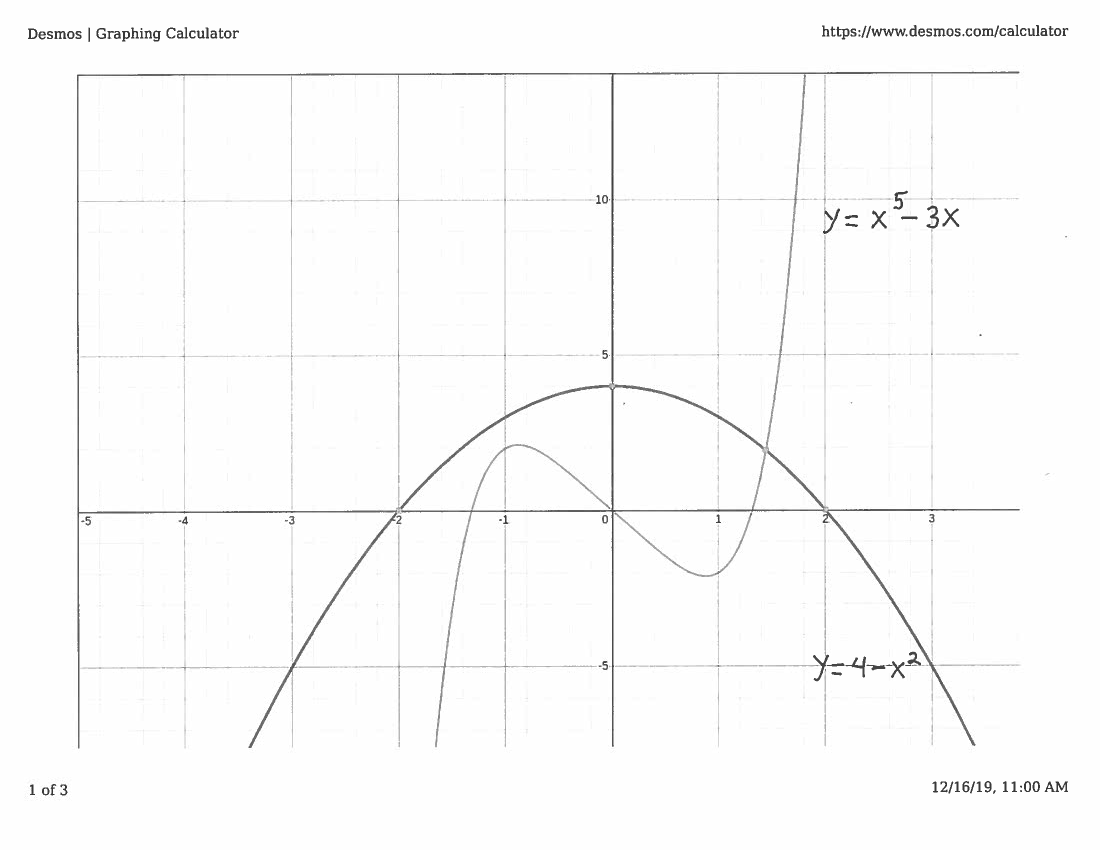SOLUTION 5: We are given the equation $$x^5-3x=4-x^2 \ \ \ \ \longrightarrow \ \ \ \ x^5+ x^2-3x-4=0$$ Let function $$f(x)=x^5+ x^2-3x-4\ \ \ \ and \ choose \ \ \ \ m=0$$ This function is continuous for all values of $x$ since it is a polynomial. To establish an appropriate interval consider the graph of this function. (Please note that the graph of the function is not necessary for a valid proof, but the graph will help us understand how to use the Intermediate Value Theorem. On many subsequent problems, we will solve the problem without using the "luxury" of a graph.)Note that $$f(0)= (0)^{5}+(0)^2-3(0)-4 =-4<0 \ \ \ \ and \ \ \ \ f(2)= (2)^{5}+(2)^2-3(2)-4=26>0$$
so that $$f(0)=-4 < m <26=f(2)$$
i.e., $m=0$ is between $f(0)$ and $f(2)$. Now choose the interval to be $\ [0, 2]$.

The assumptions of the Intermediate Value Theorem have now been met, so we can conclude that there is some number $c$ in the interval $[0, 2]$ which satisfies $$f(c)=m$$ i.e., $$c^5+c^2-3c-4=0$$ and the equation is solvable.